{{ message }}

Instantly share code, notes, and snippets.

# mGalarnyk/machineLearningWeek1Quiz2.md

Last active Jul 19, 2022
Machine Learning (Stanford) Coursera (Week 1, Quiz 2) for the github repo: https://github.com/mGalarnyk/datasciencecoursera/tree/master/Stanford_Machine_Learning

# Machine Learning Week 1 Quiz 2 (Linear Regression with One Variable) Stanford Coursera

Github repo for the Course: Stanford Machine Learning (Coursera)

## Question 1

Consider the problem of predicting how well a student does in her second year of college/university, given how well she did in her first year.

Specifically, let x be equal to the number of "A" grades (including A-. A and A+ grades) that a student receives in their first year of college (freshmen year). We would like to predict the value of y, which we define as the number of "A" grades they get in their second year (sophomore year).

Here each row is one training example. Recall that in linear regression, our hypothesis is hθ(x)=θ01x, and we use m to denote the number of training examples.

x y
5 4
3 4
0 1
4 3

For the training set given above (note that this training set may also be referenced in other questions in this quiz), what is the value of m? In the box below, please enter your answer (which should be a number between 0 and 10).

4

## Question 2

Consider the following training set of m=4 training examples:

x y
1 0.5
2 1
4 2
0 0

Consider the linear regression model hθ(x)=θ01x. What are the values of θ0 and θ1 that you would expect to obtain upon running gradient descent on this model? (Linear regression will be able to fit this data perfectly.)

• θ0=0.5,θ1=0

• θ0=0.5,θ1=0.5

• θ0=1,θ1=0.5

• θ0=0,θ1=0.5

• θ0=1,θ1=1

θ0=0,θ1=0.5

As J(θ01)=0, y = hθ(x) = θ0 + θ1x. Using any two values in the table, solve for θ0, θ1.

If you don't know how to do this, please see the following video: Solving system of linear equations

## Question 3

Suppose we set θ0=−1,θ1=0.5. What is hθ(4)?

Setting x = 4, we have hθ(x)=θ01x = -1 + (0.5)(4) = 1

## Question 4

Let f be some function so that

f(θ01) outputs a number. For this problem,

f is some arbitrary/unknown smooth function (not necessarily the

cost function of linear regression, so f may have local optima).

Suppose we use gradient descent to try to minimize f(θ01) as a function of θ0 and θ1. Which of the

following statements are true? (Check all that apply.)

• Even if the learning rate α is very large, every iteration of gradient descent will decrease the value of f(θ01).

• If the learning rate is too small, then gradient descent may take a very long time to converge.

• If θ0 and θ1 are initialized at a local minimum, then one iteration will not change their values.

• If θ0 and θ1 are initialized so that θ01, then by symmetry (because we do simultaneous updates to the two parameters), after one iteration of gradient descent, we will still have θ01.

True or False Statement Explanation
True If the learning rate is too small, then gradient descent may take a very long time to converge. If the learning rate is small, gradient descent ends up taking an extremely small step on each iteration, and therefor can take a long time to converge
True If θ0 and θ1 are initialized at a local minimum, then one iteration will not change their values. At a local minimum, the derivative (gradient) is zero, so gradient descent will not change the parameters.
False Even if the learning rate α is very large, every iteration of gradient descent will decrease the value of f(θ01). If the learning rate is too large, one step of gradient descent can actually vastly "overshoot" and actually increase the value of f(θ01).
False If θ0 and θ1 are initialized so that θ01, then by symmetry (because we do simultaneous updates to the two parameters), after one iteration of gradient descent, we will still have θ01. The updates to θ0 and θ1 are different (even though we're doing simulaneous updates), so there's no particular reason to update them to be same after one iteration of gradient descent.

Other Options:

True or False Statement Explanation
True If the first few iterations of gradient descent cause f(θ01) to increase rather than decrease, then the most likely cause is that we have set the learning rate to too large a value if alpha were small enough, then gradient descent should always successfully take a tiny small downhill and decrease f(θ01) at least a little bit. If gradient descent instead increases the objective value, that means alpha is too large (or you have a bug in your code!).
False No matter how θ0 and θ1 are initialized, so long as learning rate is sufficiently small, we can safely expect gradient descent to converge to the same solution This is not true, depending on the initial condition, gradient descent may end up at different local optima.
False Setting the learning rate to be very small is not harmful, and can only speed up the convergence of gradient descent. If the learning rate is small, gradient descent ends up taking an extremely small step on each iteration, so this would actually slow down (rather than speed up) the convergence of the algorithm.

## Question 5

Suppose that for some linear regression problem (say, predicting housing prices as in the lecture), we have some training set, and for our training set we managed to find some θ0, θ1 such that J(θ01)=0.

Which of the statements below must then be true? (Check all that apply.)

• For this to be true, we must have y(i)=0 for every value of i=1,2,…,m.

• Gradient descent is likely to get stuck at a local minimum and fail to find the global minimum.

• For this to be true, we must have θ0=0 and θ1=0 so that hθ(x)=0

• Our training set can be fit perfectly by a straight line, i.e., all of our training examples lie perfectly on some straight line.

True or False Statement Explanation
False For this to be true, we must have y(i)=0 for every value of i=1,2,…,m. So long as all of our training examples lie on a straight line, we will be able to find θ0 and θ1) so that J(θ01)=0. It is not necessary that y(i) for all our examples.
False Gradient descent is likely to get stuck at a local minimum and fail to find the global minimum. none
False For this to be true, we must have θ0=0 and θ1=0 so that hθ(x)=0 If J(θ01)=0 that means the line defined by the equation "y = θ0 + θ1x" perfectly fits all of our data. There's no particular reason to expect that the values of θ0 and θ1 that achieve this are both 0 (unless y(i)=0 for all of our training examples).
True Our training set can be fit perfectly by a straight line, i.e., all of our training examples lie perfectly on some straight line. None

Other Options:

True or False Statement Explanation
False We can perfectly predict the value of y even for new examples that we have not yet seen. (e.g., we can perfectly predict prices of even new houses that we have not yet seen.) None
False This is not possible: By the definition of J(θ01), it is not possible for there to exist θ0 and θ1 so that J(θ01)=0 None
True For these values of θ0 and θ1 that satisfy J(θ01)=0, we have that hθ(x(i))=y(i) for every training example (x(i),y(i)) None

### onur-oruc commented Jan 30, 2020 • edited

Can anyone explain the answer to question 2?
θ0=0,θ1=0.5 how it's taken

@knsaicharan

If you draw the graph according to the training set, it will be easier to write the equation: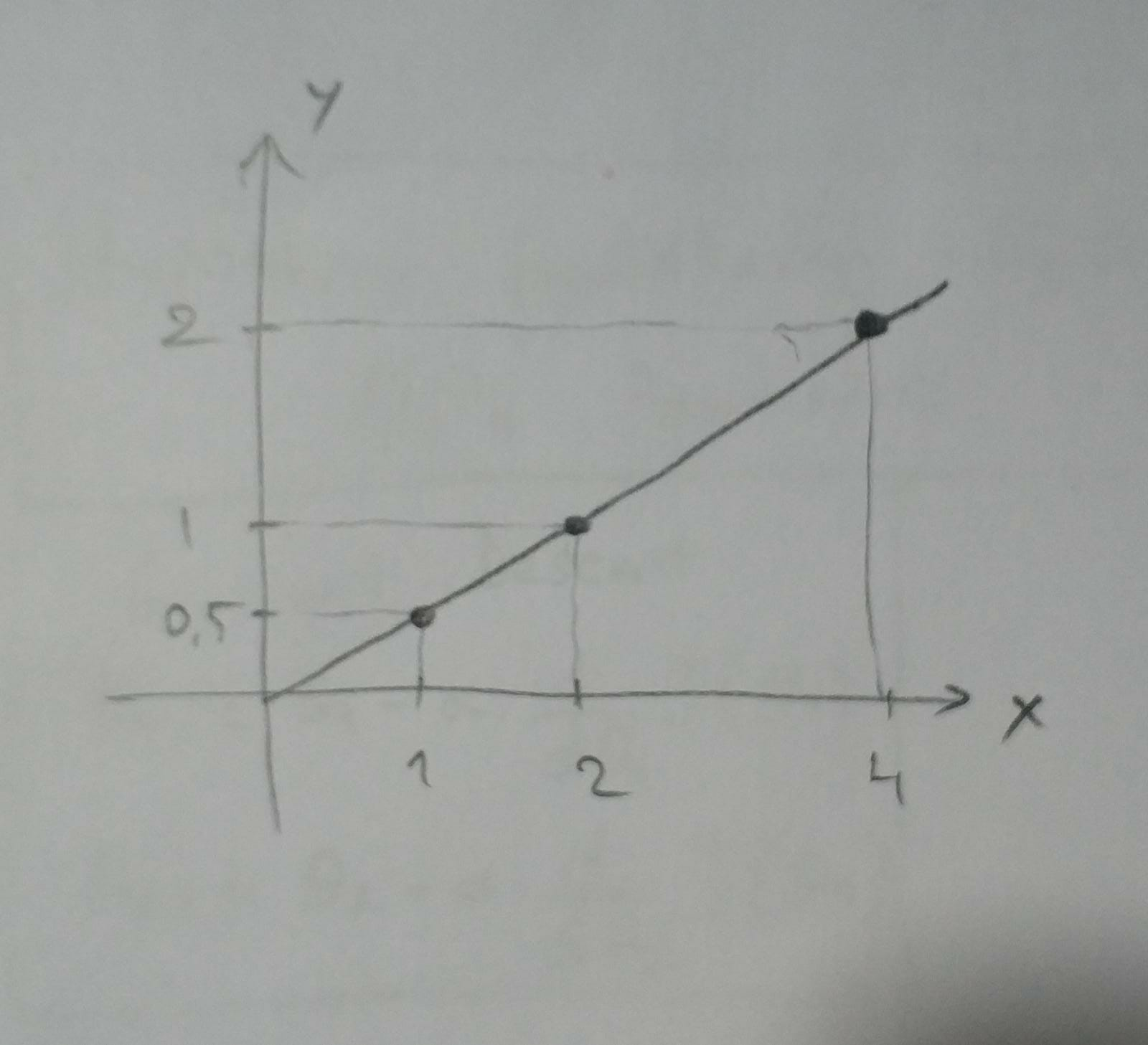### LewisLynch commented Feb 7, 2020 • edited

A good description of machine learning for student assessment. I think that it may be even more useful to include information about writing an essay. Very useful information for adapting the learning process. In my class, for example, few people knew how to write essays and used the site https://gradesfixer.com/free-essay-examples/literature/ where I could find texts on different topics to solve problems in my studies. A site with a large database of free examples of essays on literature. I hope that it will be possible to expand this database and make many additions, which relate to personal indicators of subjects with links to errors.

### ZeeshanMirza74 commented Mar 27, 2020

this is the perfect graph for ( Apply Linear Regression on the above dataset to predict the price of an unknown house ) for more info Zshani7474@gmail.com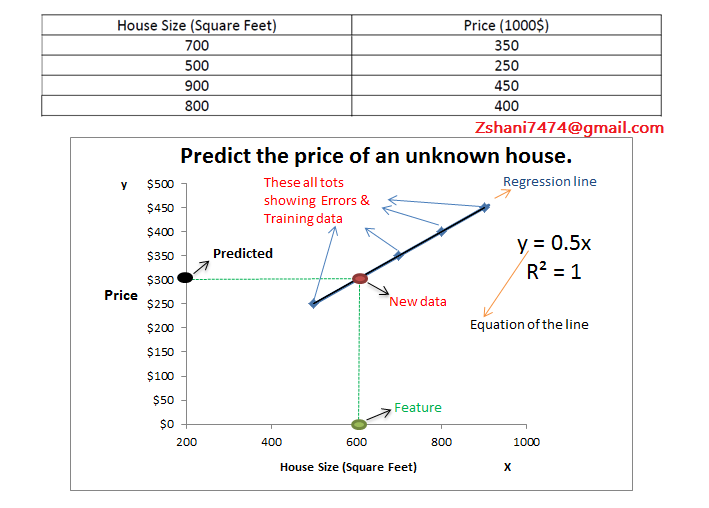### ZeeshanMirza74 commented Apr 20, 2020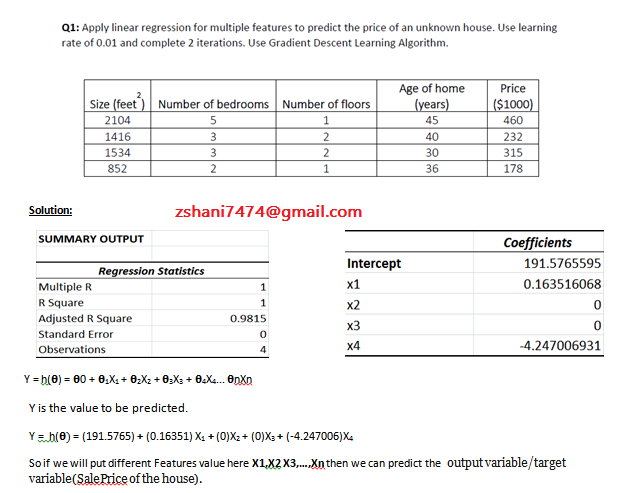this is the perfect graph for ( Apply Multiple Linear Regression on the given dataset to predict the price of an unknown house ) for more info zshani7474@gmail.com

### naklibatman commented May 1, 2020

I think 2nd option of 5th question can also be proved by saying that if their exist a global minimum then their is no chance to have any local minimum because as we have seen in lecture, the cost function of the gradient descent is always "bow shaped" which is also called "convex function" that does not contain any local minimum.

### pranavghadge commented Jun 18, 2020

Can anyone explain the answer to question 2?
θ0=0,θ1=0.5 how it's taken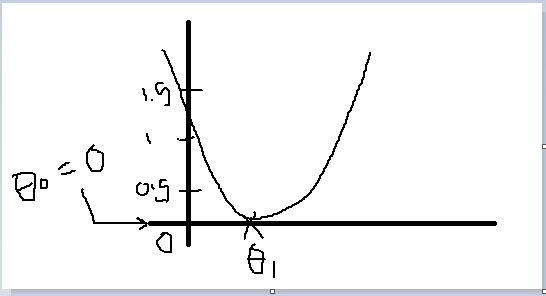when we make θ0=0 then it is mapped on the y-axis and θ1=0.5 on x-axis then it gives us the minimum cost function

### ArturoItu commented Jun 25, 2020

Great this help to improve understanding

### FalcoLambordi commented Jun 26, 2020

i really need help my dudes If someone can help me visualize this i would be more than thankfull. threw a skype or zoom call even whatsapp. I get the theory of it all. but I'm still not getting where and how do i compute this.

thanks to you guys i can pass, but i really wanna know what how to answer this questions truthfully.

great

### JakobStork commented Jul 27, 2020

Hi guys, I have been struggling with this question. Is there anyone who can help me to figure out how to solve it?

m=4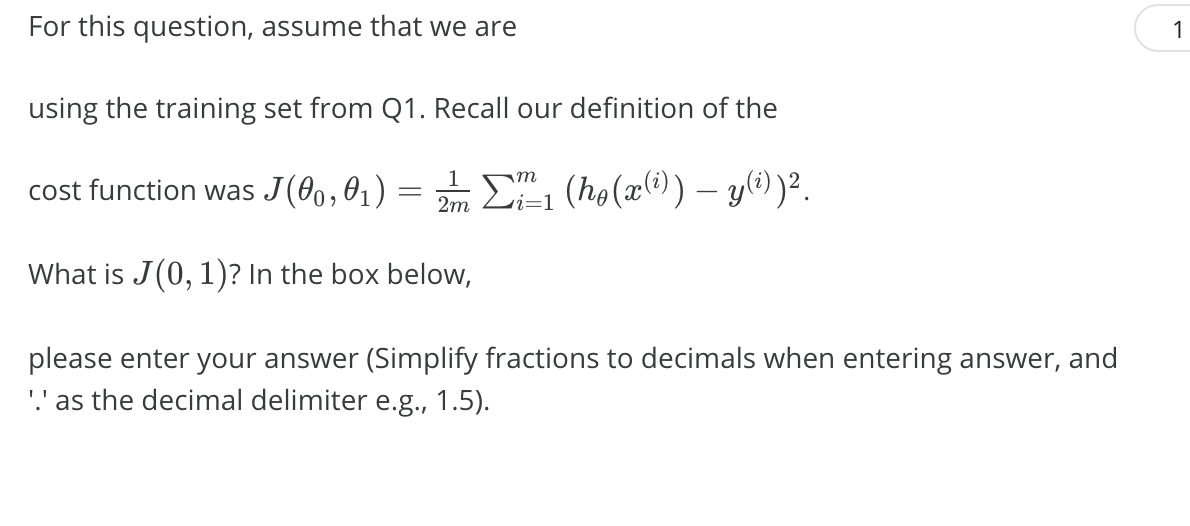### vineetsn commented Jul 29, 2020

Hi guys, I have been struggling with this question. Is there anyone who can help me to figure out how to solve it?

m=4Should be 0.

thanks bro

### NassimF commented Nov 7, 2020

Can someone explain the fourth option of question 4? I think the answer should be true according to the equations in this picture below. Since theta0=theta1, I think the equations are the same. Also, can the value of alpha be different between temp0 and temp1?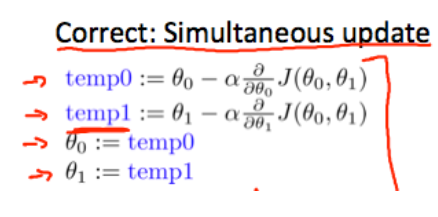### greyoreos commented Nov 9, 2020 • edited

Hi guys, I'm still confused about the solution for question 2. Does anyone have the various steps on how to solve it? I drew out the graphs and stuff but I'm still confused. I actually got the answers switched around on my own answers.
Edit: Just the steps on how to get to the answer θ0=0,θ1=0.5

### AmeenReda1 commented May 19, 2021

Hi guys, I'm still confused about the solution for question 2. Does anyone have the various steps on how to solve it? I drew out the graphs and stuff but I'm still confused. I actually got the answers switched around on my own answers.
Edit: Just the steps on how to get to the answer θ0=0,θ1=0.5

you should know that θ0 will mapped to the y-axis and θ1 mapped to the x-axis and this two values when you try to draw it on the graph it will give you the minimum cost function(Error)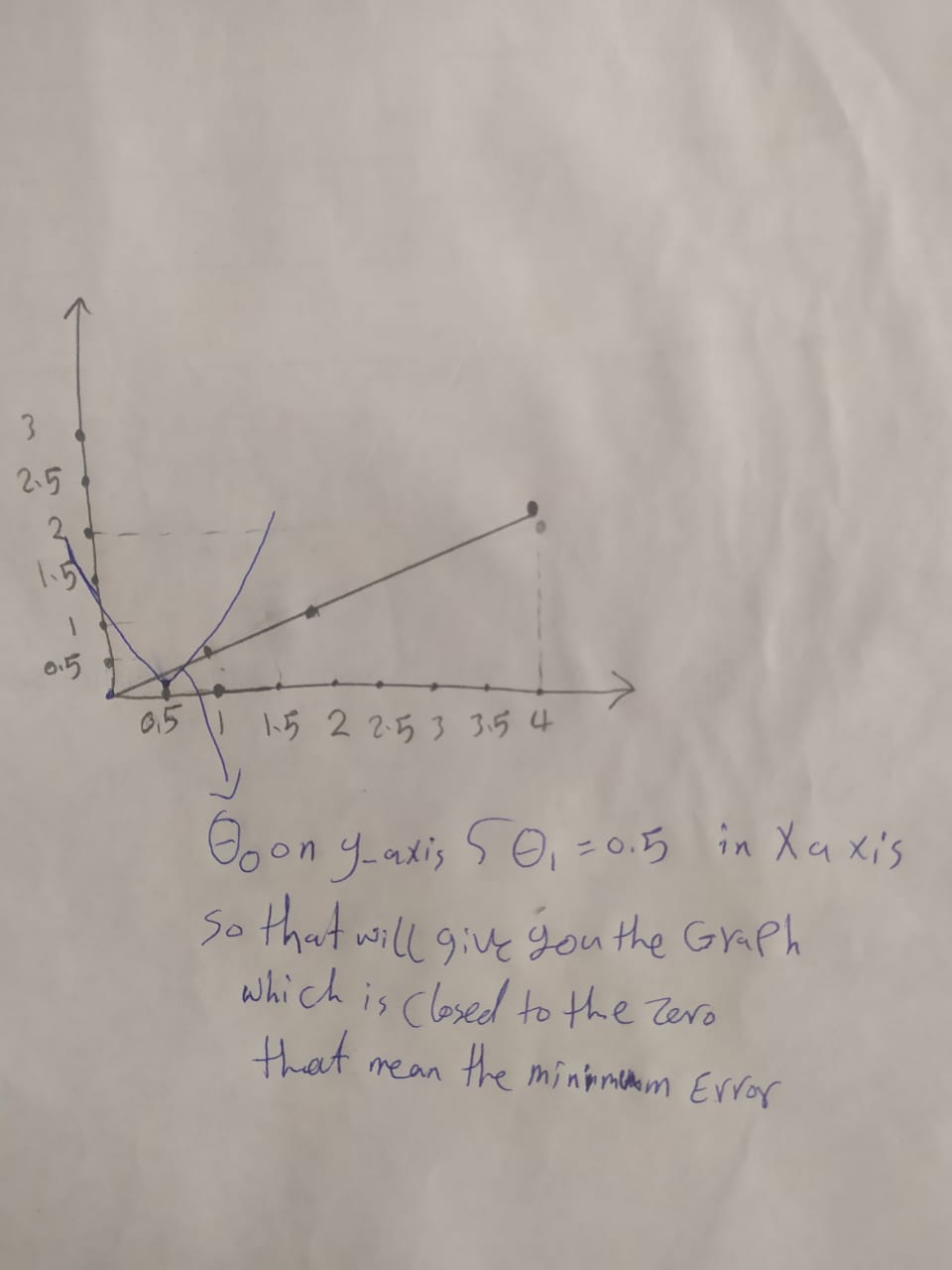### shanurrahman commented Oct 17, 2021

Can someone explain the fourth option of question 4? I think the answer should be true according to the equations in this picture below. Since theta0=theta1, I think the equations are the same. Also, can the value of alpha be different between temp0 and temp1?Hey, I am in the exact same situation. Can you point me in the direction to an explanation ?

### NassimF commented Oct 17, 2021

Can someone explain the fourth option of question 4? I think the answer should be true according to the equations in this picture below. Since theta0=theta1, I think the equations are the same. Also, can the value of alpha be different between temp0 and temp1?Hey, I am in the exact same situation. Can you point me in the direction to an explanation ?

So, I came up with an explanation, but I'm not sure if it's correct. I think the reason why temp0 and temp1 still won't be the same is that here only the "values" of theta0 and theta1 will be the same and not the variables themselves. Also, derivative doesn't care about the values and only works with the variables (eg. X, Y, theta). So the derivative of J w.r.t theta0 will be different than the derivative with respect to theta1; therefore, the value of the second term in temp0 will be different from the second term in temp1.

Hope this helps :)

### shanurrahman commented Oct 17, 2021

Can someone explain the fourth option of question 4? I think the answer should be true according to the equations in this picture below. Since theta0=theta1, I think the equations are the same. Also, can the value of alpha be different between temp0 and temp1?Hey, I am in the exact same situation. Can you point me in the direction to an explanation ?

So, I came up with an explanation, but I'm not sure if it's correct. I think the reason why temp0 and temp1 still won't be the same is that here only the "values" of theta0 and theta1 will be the same and not the variables themselves. Also, derivative doesn't care about the values and only works with the variables (eg. X, Y, theta). So the derivative of J w.r.t theta0 will be different than the derivative with respect to theta1; therefore, the value of the second term in temp0 will be different from the second term in temp1.

Hope this helps :)

It does . Thanks for the quick response...
:)

### Pongpun364 commented Oct 19, 2021

just thanks, finally found this !!!

### NassimF commented Oct 19, 2021

just thanks, finally found this !!!

Glad to be of help!

### NassimF commented Oct 19, 2021

Can someone explain the fourth option of question 4? I think the answer should be true according to the equations in this picture below. Since theta0=theta1, I think the equations are the same. Also, can the value of alpha be different between temp0 and temp1?Hey, I am in the exact same situation. Can you point me in the direction to an explanation ?

So, I came up with an explanation, but I'm not sure if it's correct. I think the reason why temp0 and temp1 still won't be the same is that here only the "values" of theta0 and theta1 will be the same and not the variables themselves. Also, derivative doesn't care about the values and only works with the variables (eg. X, Y, theta). So the derivative of J w.r.t theta0 will be different than the derivative with respect to theta1; therefore, the value of the second term in temp0 will be different from the second term in temp1.
Hope this helps :)

It does . Thanks for the quick response... :)

You're welcome!

Amazing

### Manmaya10499 commented Nov 20, 2021

Can anyone please explain to me Q-5 others - the last statement, how it's true?
"For these values of θ0 and θ1 that satisfy J(θ0,θ1)=0, we have that hθ(x(i))=y(i) for every training example (x(i),y(i))"

hθ(x(i))=y(i) can't be the same for all the training examples right. Because some of the points will not fall on the line hθ(x(i)).

### NassimF commented Nov 20, 2021

Can anyone please explain to me Q-5 others - the last statement, how it's true? "For these values of θ0 and θ1 that satisfy J(θ0,θ1)=0, we have that hθ(x(i))=y(i) for every training example (x(i),y(i))"

hθ(x(i))=y(i) can't be the same for all the training examples right. Because some of the points will not fall on the line hθ(x(i)).

I didn't get your question exactly, but I'm gonna discuss it from two points of view:
First, I think we can say it is because the question is 'supposing' that h(x(i))=y(i). In a real-world situation this will probably never happen but here we have a hypothetical situation. So if we 'imagine' that we have found the perfect theta0 and theta1 so that our predictions are 'exactly' the same as the actual value, then it mean that our line perfectly fits all the points.

Second, if you thought that the prediction and actual value are gonna be the same 'shared' value for all data points, then this is not true. For example, the question doesn't mean that h(x(i))=y(i)=4 for all data points. It just means that whatever unique value h(x(i)) has, is the same as y(i) 'for that unique data point'. So for one data point we can have 5=5 and for the other 4=4.

I hope I captured what you meant.

### H1manshus0ni commented Feb 5, 2022

my answer is 73.35 somthing lol . h= 0+X;

please tell me what mistake im doing

### phongvu009 commented Apr 11, 2022 • edited

the h(x) = 1*x + 0
for each x
you will get h(x)
calculate the error between h(x) and y

get total sum of square error in the formula of J to get the result

to join this conversation on GitHub. Already have an account? Sign in to comment